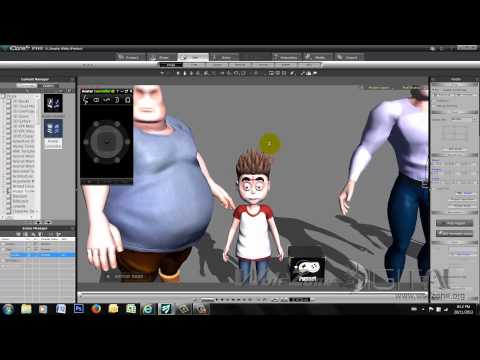# Samples

Below are some sample client videos we’ve created.

Corporate Holiday Card
```/* <![CDATA[ */ jQuery(document).ready(function(\$){ \$(function(){ var width = \$(window).innerWidth(); var setwidth = 560; var ratio = 0.5625; var height = 315; var link = 'https://www.youtube.com/watch?v=zExLXLy5WUI&amp;rel=0&width=560&height=315'; if(width < setwidth) { height = Math.floor(width * 0.5625); //console.log("device width "+width+", set width "+560+", ratio "+0.5625+", new height "+ height); var new_url = wpvl_paramReplace('width', link, width); var new_url = wpvl_paramReplace('height', new_url, height); \$("a#628a636d4240e").attr('href', new_url); //console.log(new_url); } }); }); /* ]]&gt; */ ```
Product Visual
```/* <![CDATA[ */ jQuery(document).ready(function(\$){ \$(function(){ var width = \$(window).innerWidth(); var setwidth = 560; var ratio = 0.5625; var height = 315; var link = 'https://www.youtube.com/watch?v=hD2T-e7WKgo&amp;rel=0&width=560&height=315'; if(width < setwidth) { height = Math.floor(width * 0.5625); //console.log("device width "+width+", set width "+560+", ratio "+0.5625+", new height "+ height); var new_url = wpvl_paramReplace('width', link, width); var new_url = wpvl_paramReplace('height', new_url, height); \$("a#628a636d425d5").attr('href', new_url); //console.log(new_url); } }); }); /* ]]&gt; */ ```
Corporate Profile
```/* <![CDATA[ */ jQuery(document).ready(function(\$){ \$(function(){ var width = \$(window).innerWidth(); var setwidth = 560; var ratio = 0.5625; var height = 315; var link = 'https://www.youtube.com/watch?v=ZJlTW-adds4&amp;rel=0&width=560&height=315'; if(width < setwidth) { height = Math.floor(width * 0.5625); //console.log("device width "+width+", set width "+560+", ratio "+0.5625+", new height "+ height); var new_url = wpvl_paramReplace('width', link, width); var new_url = wpvl_paramReplace('height', new_url, height); \$("a#628a636d4278a").attr('href', new_url); //console.log(new_url); } }); }); /* ]]&gt; */ ```
Product Review
```/* <![CDATA[ */ jQuery(document).ready(function(\$){ \$(function(){ var width = \$(window).innerWidth(); var setwidth = 560; var ratio = 0.5625; var height = 315; var link = 'https://www.youtube.com/watch?v=rb8cgbC69c8&amp;rel=0&width=560&height=315'; if(width < setwidth) { height = Math.floor(width * 0.5625); //console.log("device width "+width+", set width "+560+", ratio "+0.5625+", new height "+ height); var new_url = wpvl_paramReplace('width', link, width); var new_url = wpvl_paramReplace('height', new_url, height); \$("a#628a636d42a5e").attr('href', new_url); //console.log(new_url); } }); }); /* ]]&gt; */ ```

Training Scenario Sample
```/* <![CDATA[ */ jQuery(document).ready(function(\$){ \$(function(){ var width = \$(window).innerWidth(); var setwidth = 560; var ratio = 0.5625; var height = 315; var link = 'https://www.youtube.com/watch?v=BOkEPKAKke8&amp;rel=0&width=560&height=315'; if(width < setwidth) { height = Math.floor(width * 0.5625); //console.log("device width "+width+", set width "+560+", ratio "+0.5625+", new height "+ height); var new_url = wpvl_paramReplace('width', link, width); var new_url = wpvl_paramReplace('height', new_url, height); \$("a#628a636d42bbd").attr('href', new_url); //console.log(new_url); } }); }); /* ]]&gt; */ ```
```/* <![CDATA[ */ jQuery(document).ready(function(\$){ \$(function(){ var width = \$(window).innerWidth(); var setwidth = 560; var ratio = 0.5625; var height = 315; var link = 'https://www.youtube.com/watch?v=_TS5qdWFv00&amp;rel=0&width=560&height=315'; if(width < setwidth) { height = Math.floor(width * 0.5625); //console.log("device width "+width+", set width "+560+", ratio "+0.5625+", new height "+ height); var new_url = wpvl_paramReplace('width', link, width); var new_url = wpvl_paramReplace('height', new_url, height); \$("a#628a636d42d50").attr('href', new_url); //console.log(new_url); } }); }); /* ]]&gt; */ ```